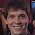## Sunday, 3 May 2009

### Levenshtein Distance in Clojure (II)

In my last post, I looked at the Levenshtein Distance and showed that the time complexity of the algorithm (when naively implemented) is exponential. In this post I'll show how we can improve this time complexity of this algorithm with a small series of changes.

Dynamic programming is a technique for solving problems which consist of overlapping subproblems. The Levenshtein algorithm is perfect for this kind of problem as the final solution is the sum of the little solutions. Imperative languages will often solve this using a M x N matrix, where each item represents the cost taken to convert an item so far. This is expanded in much more detail on Wikipeda. This solution is nice, and computationally cheap, but it's not faithful to the definition of the problem. It's an example of the impedance mismatch between writing software and writing specifications for a problem (see previous post about Intentional Software for one approach to minimizing this mismatch).

So how can we keep faithful to the original specification without having exponential performance cost? Memoization is a technique for caching the results of function calls. Functional programming languages are inherently suitable for memoization, as every pure function will, for the same arguments, yield the same result. Clojure has direct support for memoization via `memoize`. If we were dealing with a simple example where the function body didn't refer to the function name, we could just use memoize directly, but Levenshtein distance refers to itself three times. There are a number of options here, we could either convert to a tail recursive function and recur will solve the problem or we could use a forward declaration (thanks for #Clojure for pointing that one out, you don't want to know what my plan C was!).

Transforming the algorithm to be tail recursive is going to be painful and radically change the way the code looks. So let's go for the forward declaration approach. We declare `levenshtein-distance-fast` up front, write the declaration of `levenshtein-distance` referring to the fast implementation (as yet unbound) and finally declare `levenshtein-distance-fast` as the memoized version of `levenshtein-distance`.

`(declare levenshtein-distance-fast)(defn levenshtein-distance-int  "Calculates the edit-distance between two sequences"  [seq1 seq2]  (cond    (empty? seq1) (count seq2)    (empty? seq2) (count seq1)    :else (min           (+ (cost (first seq1) (first seq2)) (levenshtein-distance-fast (rest seq1) (rest seq2))) ;; substitution           (inc (levenshtein-distance-fast (rest seq1) seq2))    ;; insertion           (inc (levenshtein-distance-fast seq1 (rest seq2)))))) ;; deletion(def levenshtein-distance-fast (memoize levenshtein-distance))`

This makes a huge difference in performance as you can see below:

`  user> (time (levenshtein-distance-fast "abcdefghi" "hijklmnop"))  "Elapsed time: 0.191776 msecs"  user> (time (levenshtein-distance-fast "abcdefghi" "hijklmnop"))  "Elapsed time: 0.081372 msecs"  user> (time (levenshtein-distance-slow "abcdefghi" "hijklmnop"))  "Elapsed time: 668.713644 msecs"  9`

This (probably!) gives the same complexity class as a implementation using dynamic programming. However, it still has one big problem. It isn't tail recursive and hence it won't work for large very length sequences passed as arguments. Altering the definition to use tail recursion is not something I fancy doing!

#### 6 comments:

1.Found via a vanity search that someone said "bottom of the third paragraph there sure seems to imply that recur uses memoize"

Definitely didn't mean to imply that! recur is just a way of recursing from the tail position without blowing the stack. memoize is caching function return values such that a subsequent invocation with the same arguments gives the result instantly. There's no relationship between the two!

2.I was trying to do a very similar thing, declare was just what I was after, thanks!

3.This implementation is clever, but looks like a memory devourer. Using this over fairly large datasets, the cost of memoizing every subsequence (especially if you're using a seq of some other object besides char, and thus have a larger "alphabet") becomes crazy.

4.Cory - this is definitely a memory devourer! I thought it was just an interesting way of keeping the code looking neat and getting the performance. Definitely not production code!

5.Looks like you didn't include a definition of cost here. You must have had your version from the previous post defined in your REPL, yes?

(defn cost [a b]
(if (= a b) 0 1))

6.Ethan, well spotted. Almost certainly left over from the REPL!